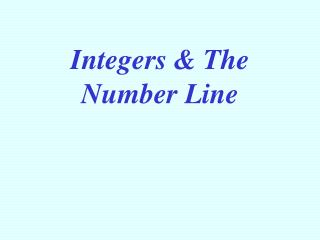DownloadDownload PresentationIntegers & The Number Line

Integers & The Number Line

Télécharger la présentationIntegers & The Number Line

- - - - - - - - - - - - - - - - - - - - - - - - - - - E N D - - - - - - - - - - - - - - - - - - - - - - - - - - -
Presentation Transcript

1. Integers & The Number Line

2. -5 0 5 -10 10 Number Lines • A number line is a line with marks on it that are placed at equal distances apart. • One mark on the number line is usually labeled zero and then each successive mark to the left or to the right of the zero represents a particular unit such as 1 or ½. • On the number line above, each small mark represents ½ unit and the larger marks represent 1 unit.

3. -5 0 5 -10 10 Number Lines Number lines can be used to represent: • Whole numbers – the set {0, 1, 2, 3, …} • Positive numbers – any number that is greater than zero • Negative numbers – any number that is less than zero • Integers – the set of numbers represented as {…, -3, -2, -1, 0, 1, 2, 3, …} The arrows at the ends of the number line show that the number line continues in both directions without ending.

4. -5 0 5 -10 10 Graphing on Number Lines A number can be graphed on a number line by placing a point at the appropriate position on the number line. Example • {4} (blue point) • {integers between –10 and –5} (purple)

5. -5 0 5 -10 10 Graphing on Number Lines • Name the set of numbers that is graphed. {-8, -4, 1, 5, 8} {-8, -4, 1, 5, 8}

6. -5 0 5 -10 10 Moving on Number Lines • Movement to the right on the number line is in the positive direction (increasing). Do this to add a positive #. • Movement to the left on the number line is in the negative direction (decreasing). Do this to add a negative #. • Make the following moves on the number line. Start at 5 and move left 7 integers. • Where did you stop? -2 How can we represent this mathematically? 5 + (-7) = -2

7. 1) Graph these pairs of numbers on a number line. Write two inequalities comparing the two numbers. a) -2, 7 b) -9, -4 c) 3, 8 2) Find each sum using a number line. Place the 1st # on a # line, then move to the right or left. a) 3 + 7 b) -1 + (-7) c) -4 + 12 d) -9 + 5 e) -6 + 6 You Try It!

8. -5 -5 0 0 5 5 -10 -10 10 10 Problems 1 & 2

9. -5 -5 0 0 5 5 -10 -10 10 10 Problems 3 & 4 Show 3 + 7 using the number line. Start at 3 and move 7 places to the right. 3 + 7 = 10 Show -1 +(-7) using a number line. Start at -1 and move 7 places to the left. -1 + (-7) = -8

10. -5 -5 0 0 5 5 -10 -10 10 10 Problems 5 & 6 Show –9 + 5 using the number line. Start at –9 and move 5 places to the right. –9 + 5 = –4 Show -6 + 6 using a number line. Start at -6 and move 6 places to the left. -6 + 6 = 0